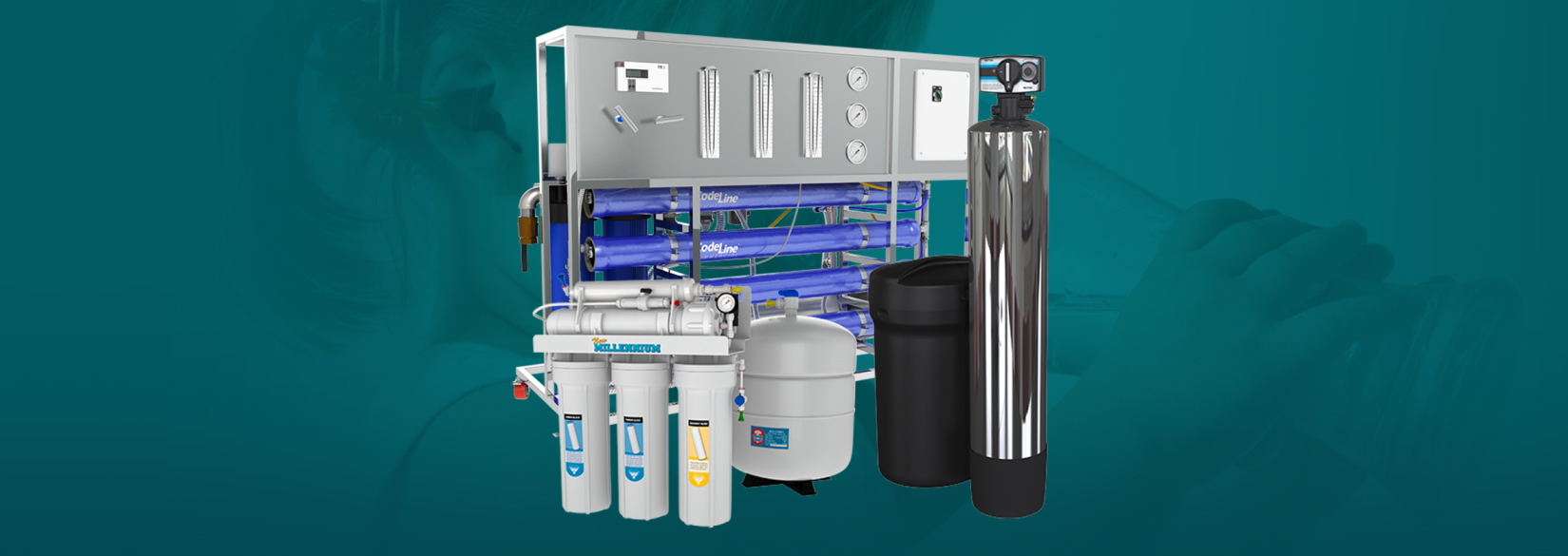# Case Study

## Waterworld MoroccoWaterworld Morocco takes pride in customer service by offering a complete line of
water treatment goods and supporting them with technical support.## Problem

Here is where you add the problems. Here is where you add the problems. Here is where you add the problems. Here is where you add the problems. Here is where you add the problems. Here is where you add the problems. Here is where you add the problems.## Solution

Here is where you add the problems. Here is where you add the problems. Here is where you add the problems. Here is where you add the problems. Here is where you add the problems. Here is where you add the problems. Here is where you add the problems.## Outcome

Here is where you add the problems. Here is where you add the problems. Here is where you add the problems. Here is where you add the problems. Here is where you add the problems. Here is where you add the problems. Here is where you add the problems.## Website Design and Development

You can add the contents for this project here.

### Logo

You can add the contents for this project here. You can add the contents for this project here. You can add the contents for this project here. You can add the contents for this project here. You can add the contents for this project here.